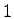## 1. 1. 8 Mathematical Solution

The coefficients of this solution vector are multiplied with the basis functions and the sum of the weighted basis functions is the appropriate solution of the discretized problem. If functions with local support with a maximum valueare used, the stored numbers can be interpreted as function values in the points where the respective shape function has its maximum. However, many methods such as the boundary element method show that this is not the case in general. Many methods exist which do not use function spaces with this special property and therefore, cannot be interpreted in this way.

It has to be stated that once the function space is determined in the process of functional discretization, there is no way of changing the shape of the base functions due to e.g. physical or mathematical reasons after the calculation is performed.

Even though this seems to be clear from the mathematical point of view, many post-processing tools, which are not directly involved in the discretization process, provide different means of obtaining the original function by different interpolation methods. In order to obtain the correct function the interpolation method has to be used which also was used in the discretization step. This is of special relevance, if differential terms such as gradients have to be evaluated in ``non-grid'' points.

Michael 2008-01-16